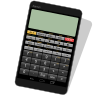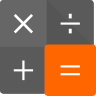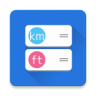##### Panecal scientific calculator

Panecal scientific calculator can display and correct mathematical formulas such as editing and recalculating calculation formulas entered in the past, as well as the capability to use variable memory to only change specific values and do calculations.##### Calculator PanecalST

The design of the PanecalST is very simple and basic calculator, but the PanecalST has some powerful and useful functions such as cursor formulas editing, calculation of value including tax, percent, last answer, answer history, re-play formulas.##### ShapeInfo

The ShapeInfo is useful application for calculating shape lengths, angles and area. You can get some calculated lengths and angles of the shape from choosing and entering values in the boxes upon the orange points.##### Unit Converter

This is unit converter application. The cursor is also displayed by numerical input by the numeric keypad. The result of the conversion value can be transferred to the clipboard or “Panecal scientific calculator”.